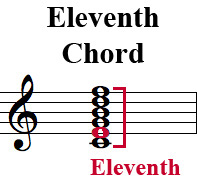# eleventh chord

[English]

A chord having up to six notes, the interval between the root note and the highest note being the interval of an eleventh. The other notes in the chord would be the root, third, fifth, seventh, and ninth. In the "Cchord below, the root is C, the third is E, the fifth is G, the seventh is B, the ninth is D, and the eleventh is F. This chord is typically performed without the third (in this example the note E) due to the dissonance with the eleventh (in this example the note F).

### Example